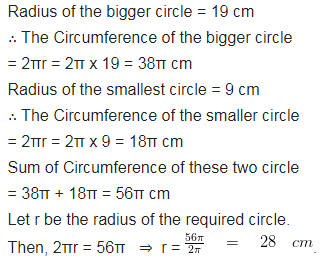# The radii of the two circles are 19 cm and 9 cm respectively

The radii of the two circles are 19 cm and 9 cm respectively. Find the radius of the circle which has a circumference equal to the sum of the circumferences of the two circles.